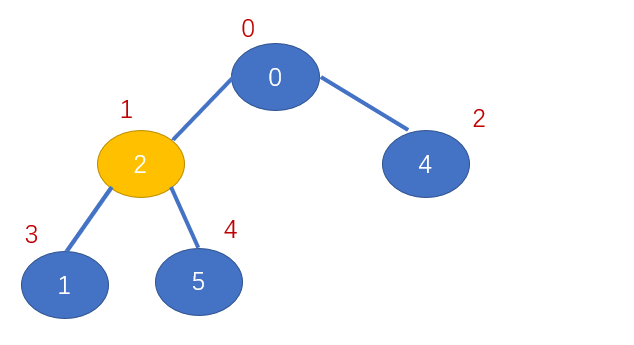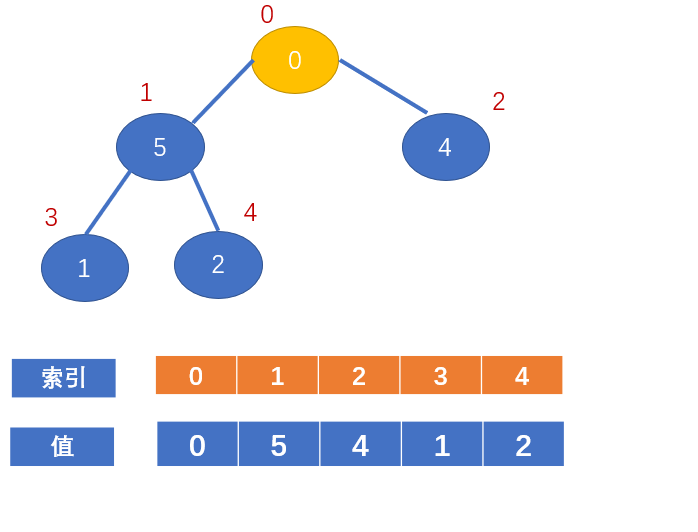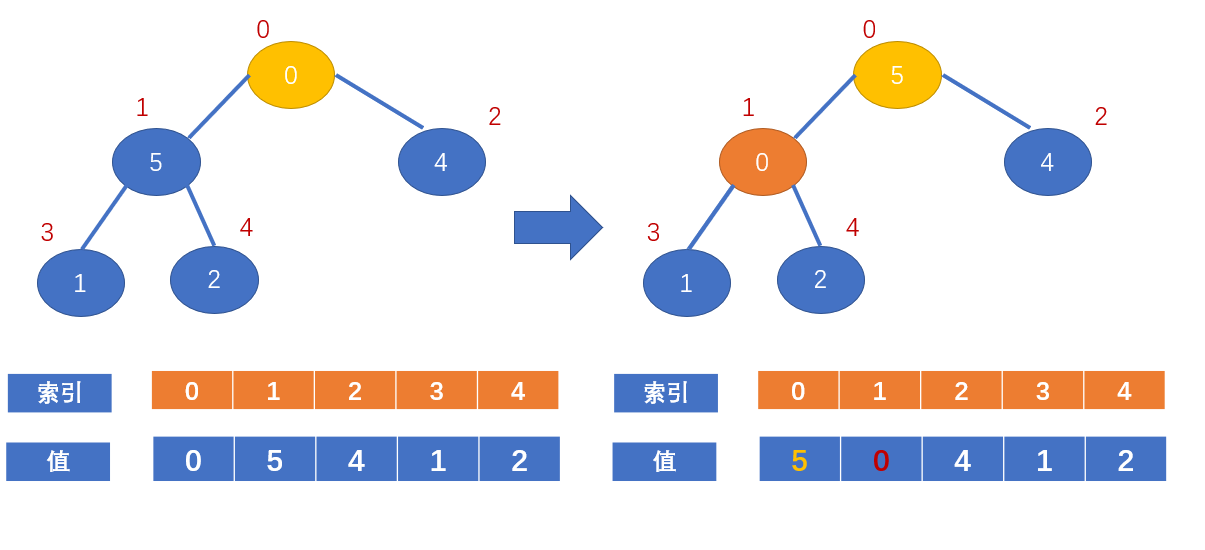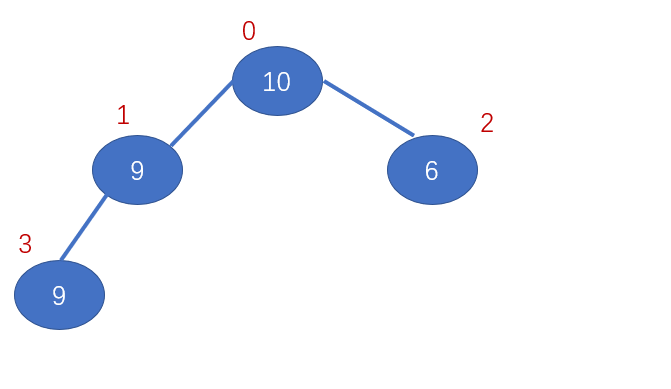# 详解堆排序

2020年5月19日15:22:43评论98阅读模式

## 什么是堆

### 堆特点

arr[i] >= arr[ 2i + 1] && arr[ i ] >= arr[ 2i + 2];

arr[i] <= arr[ 2i + 1] && arr[ i ] <= arr[ 2i + 2];文章源自随机的未知-https://sjdwz.com/11143.html

## 堆排序基本思想

1、首先将待排序序列构建成一个大顶堆(存入数组中)，那么这时，整个序列的最大值就是堆顶的根节点；
2、将堆顶元素与最后一个元素交换，那么末尾元素就存入了最大值；
3、将剩余的 n - 1个元素重新构建成一个大顶堆，重复上面的操作；

## 举例

### 构建堆文章源自随机的未知-https://sjdwz.com/11143.html## 代码

`````` /**
* author:微信公众号:code随笔
* @param arr 待排序的数组
* @param i   表示等待调整的哪个非叶子节点的索引
* @param length 待调整长度
*/
public static void adjustHeap(int arr[],int i,int length){
//非叶子节点的值
int notLeafNodeVal = arr[i];
//k的初始值为当前非叶子节点的左孩子节点的索引
//k = 2 * k + 1表示再往左子节点找
for(int k = i * 2 + 1;k<length;k=2 *k + 1){
//如果k + 1还在待调整的长度内，且右子树的值大于等于左子树的值
//将k++，此时为当前节点的右孩子节点的索引
if(k+1<length && arr[k] < arr[k+1]){
k++;
}
//如果孩子节点大于当前非叶子节点
if(arr[k] > notLeafNodeVal){
arr[i] = arr[k];//将当前节点赋值为孩子节点的值
i = k;//将i赋值为孩子节点的值，再看其孩子节点是否有比它大的
}else{
break;//能够break的保证是，我们是从左至右，从下到上进行调整的
//只要上面的不大于，下面的必不大于
}
}
//循环结束后，将i索引处的节点赋值为之前存的那个非叶子节点的值
arr[i] = notLeafNodeVal;
}
``````

``````//堆排序方法
public static void heapSort(int arr[]){
//进行第一次调整
for(int i=arr.length/2 - 1;i>=0;i--){
}

for(int j=arr.length - 1;j>0;j--){
//进行交换
int temp = arr[j];
arr[j] = arr;
arr = temp;
//调整长度为j的那些
//这里为什么填0呢
//因为我们第一次调整的时候从左到右，从下到上调整的；
//交换时只是变动了堆顶元素和末尾元素
//末尾元素我们不用去管，因为已经是之前长度最大的了
//只需要把当前堆顶元素找到合适的位置即可
}
}
``````

``````import java.util.Arrays;

public class Solution {
public static void main(String[] args) {

int [] arr = new int[]{0 , 2,  4,  1 , 5};
heapSort(arr);
System.out.println(Arrays.toString(arr));

}
//堆排序方法
public static void heapSort(int arr[]){
//进行第一次调整
for(int i=arr.length/2 - 1;i>=0;i--){
}

for(int j=arr.length - 1;j>0;j--){
//进行交换
int temp = arr[j];
arr[j] = arr;
arr = temp;
//调整长度为j的那些
//这里为什么填0呢
//因为我们第一次调整的时候从左到右，从下到上调整的；
//交换时只是变动了堆顶元素和末尾元素
//末尾元素我们不用去管，因为已经是之前长度最大的了
//只需要把当前堆顶元素找到合适的位置即可
}
}
/**
* author:微信公众号:code随笔
* @param arr 待排序的数组
* @param i   表示等待调整的哪个非叶子节点的索引
* @param length 待调整长度
*/
public static void adjustHeap(int arr[],int i,int length){
//非叶子节点的值
int notLeafNodeVal = arr[i];
//k的初始值为当前非叶子节点的左孩子节点的索引
//k = 2 * k + 1表示再往左子节点找
for(int k = i * 2 + 1;k<length;k=2 *k + 1){
//如果k + 1还在待调整的长度内，且右子树的值大于等于左子树的值
//将k++，此时为当前节点的右孩子节点的索引
if(k+1<length && arr[k] < arr[k+1]){
k++;
}
//如果孩子节点大于当前非叶子节点
if(arr[k] > notLeafNodeVal){
arr[i] = arr[k];//将当前节点赋值为孩子节点的值
i = k;//将i赋值为孩子节点的值，再看其孩子节点是否有比它大的
}else{
break;//能够break的保证是，我们是从左至右，从下到上进行调整的
//只要上面的不大于，下面的必不大于
}
}
//循环结束后，将i索引处的节点赋值为之前存的那个非叶子节点的值
arr[i] = notLeafNodeVal;
}
}
``````

## 稳定性## 欢迎关注文章源自随机的未知-https://sjdwz.com/11143.html

• 本文由 发表于 2020年5月19日15:22:43
• 转载请注明：来源：随机的未知 本文链接https://sjdwz.com/11143.html